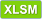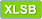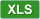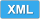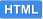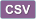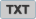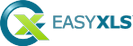# How to convert XML spreadsheet file to Excel in C++.NET

 View Excel fileCopy code ``````/*---------------------------------------------------------------------------- | Tutorial 41 | | This tutorial shows how to convert XML spreadsheet to Excel in C++.NET. The | XML Spreadsheet generated by Tutorial 32 is imported, some data is modified | and after that is exported as Excel file. -----------------------------------------------------------------------------*/ #include "stdafx.h" using namespace System; using namespace EasyXLS; using namespace System::Data; using namespace System::IO; int main() { Console::WriteLine("Tutorial 41\n----------\n"); // Create an instance of the class used to import/export Excel files ExcelDocument ^workbook = gcnew ExcelDocument(); // Import XML Spreadsheet file Console::WriteLine("Reading file C:\\Samples\\Tutorial32.xml.\n"); if (workbook->easy_LoadXMLSpreadsheetFile("C:\\Samples\\Tutorial32.xml")) { // Get the table of data from the second sheet and add some data in cells (optional step) ExcelWorksheet ^xlsSecondTab = safe_cast(workbook->easy_getSheetAt(1)); ExcelTable ^xlsTable = xlsSecondTab->easy_getExcelTable(); xlsTable->easy_getCell("A1")->setValue("Data added by Tutorial41"); for (int column=0; column<5; column++) { xlsTable->easy_getCell(1, column)->setValue(String::Concat("Data ", (column + 1).ToString())); } // Export Excel file Console::WriteLine("Writing file C:\\Samples\\Tutorial41 - convert XML spreadsheet to Excel.xlsx."); workbook->easy_WriteXLSXFile("C:\\Samples\\Tutorial41 - convert XML spreadsheet to Excel.xlsx"); // Confirm conversion of XML Spreadsheet to Excel String ^sError = workbook->easy_getError(); if (sError->Equals("")) Console::Write("\nFile successfully created. Press Enter to Exit..."); else Console::Write(String::Concat("\nError encountered: ", sError, "\nPress Enter to Exit...")); } else { Console::WriteLine(String::Concat("\nError reading file C:\\Samples\\Tutorial32.xml \n", workbook->easy_getError(), "\nPress Enter to Exit...")); } // Dispose memory delete workbook; Console::ReadLine(); return 0; } ``````

C++ without .NET Framework
This tutorial is valid for C++ applications that can use .NET Framework. For applications that does not have .NET Framework integrated, a similar code sample is available.

#### EasyXLS Excel libraries: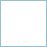##### Excel Library for C++.NET
full .NET version to import, export or convert Excel files#### File formats:

MS Excel 97 - 2003
MS Excel 2007 - 2010
MS Excel 2013
MS Excel 2016
MS Excel 2019